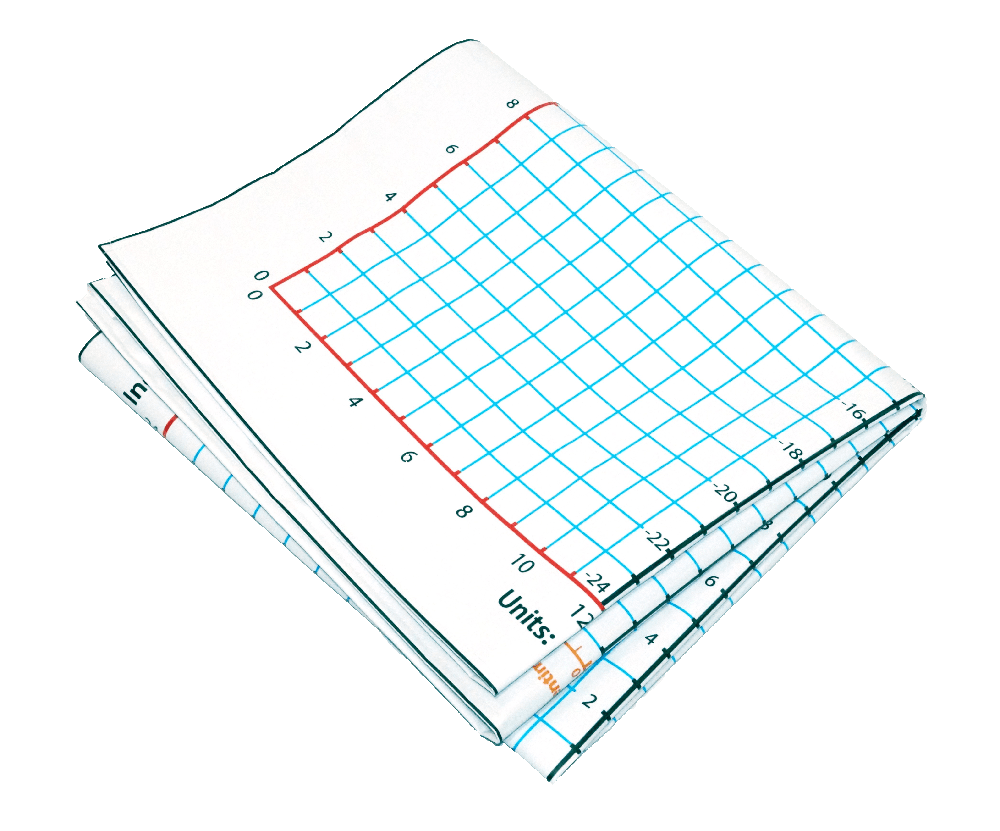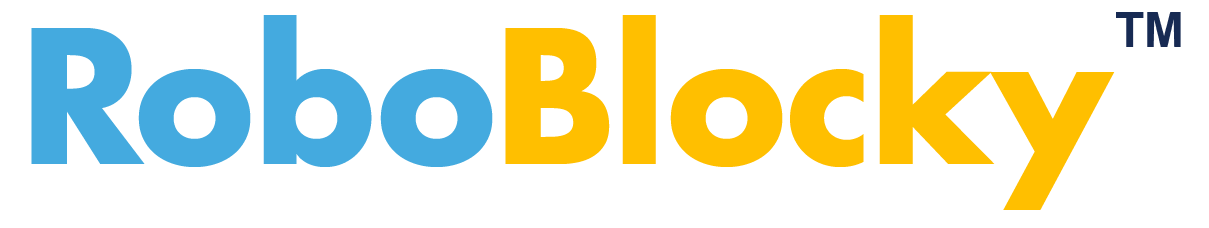Learning Math with Coding and Robotics
Algebra 1 with RoboBlocky (grade 8+)

Try these engaging robotics activities designed to help learn coding while practicing Algebra 1 concepts. Use RoboBlocky to control a virtual robot, hardware Linkbot, or both!

 Modeling Linear Relations 1 of Velocity and Time Modeling Linear Relations 2 in the Coordinate Plane Modeling Linear Relations 3 in the Coordinate Plane Graphing Linear Equations with Robots 1: Intercepts Graphing Linear Equations with Robots 2: Slope & y-intercept Graphing Systems of Linear Equations with Robots P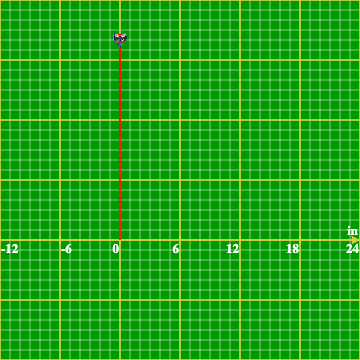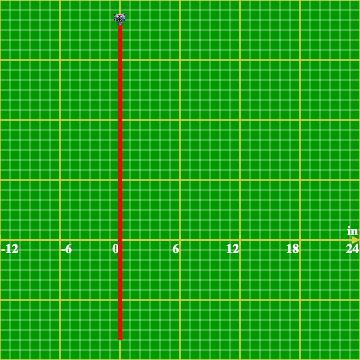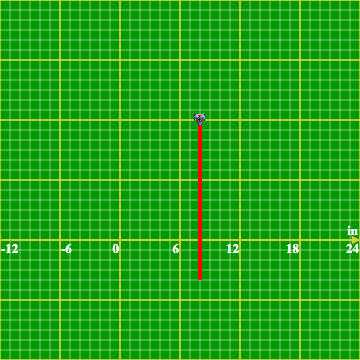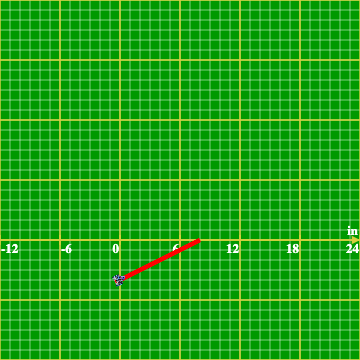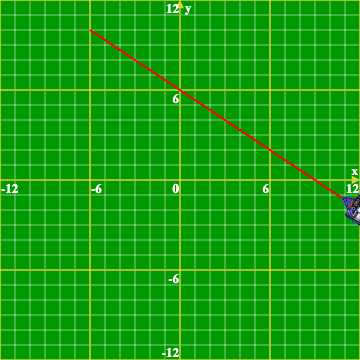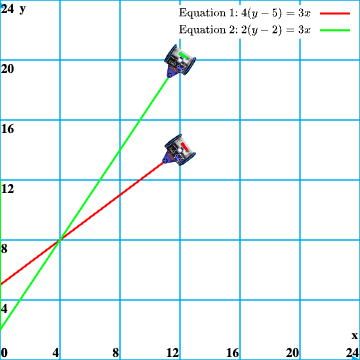Graphing Systems of Linear Inequalities with Robots Geometry: Area of Rectangle in Coordinate Plane Geometry: Area of Triangle in Coordinate Plane Model & Calculate the Average Speed of a Robot in the Coordinate Plane Graph Cake Sales Equations - Solving for Missing Values and Modeling their Solution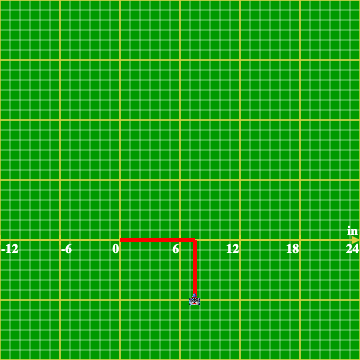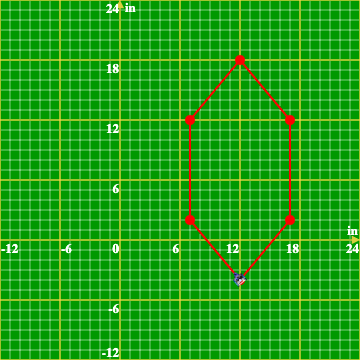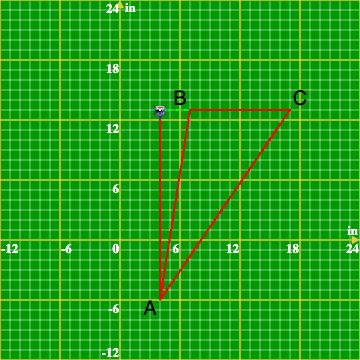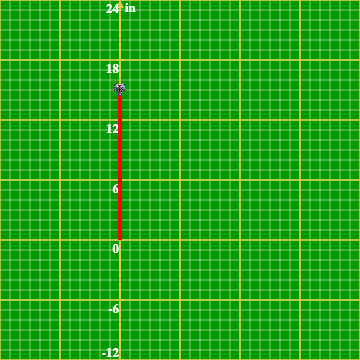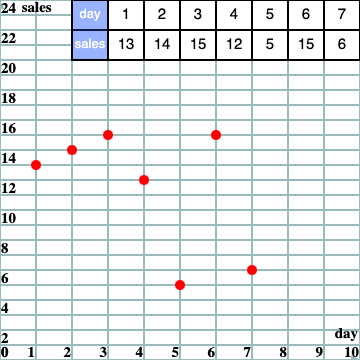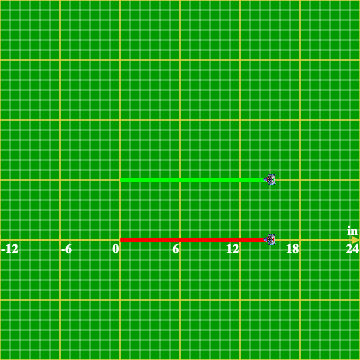Initial Position - Modeling in the Coordinate Plane Initial Position and Initial Angle - Modeling in the Coordinate Plane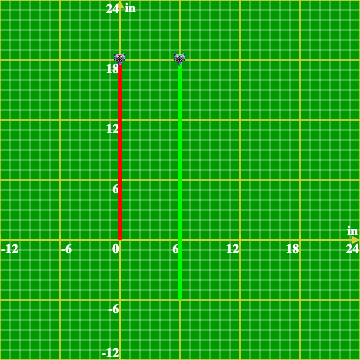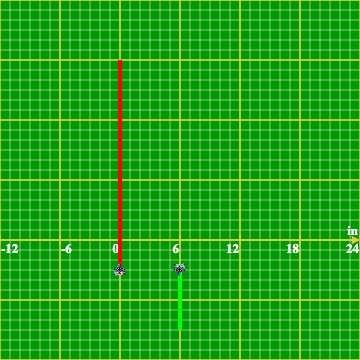### Hardware (optional):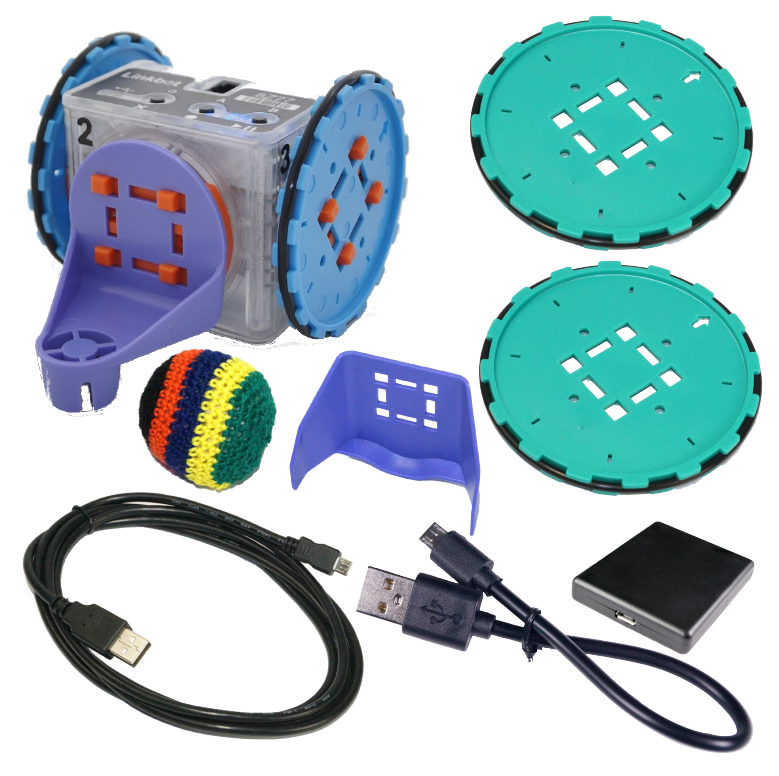Activity mats: